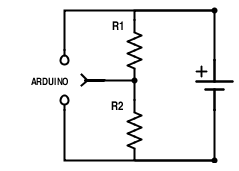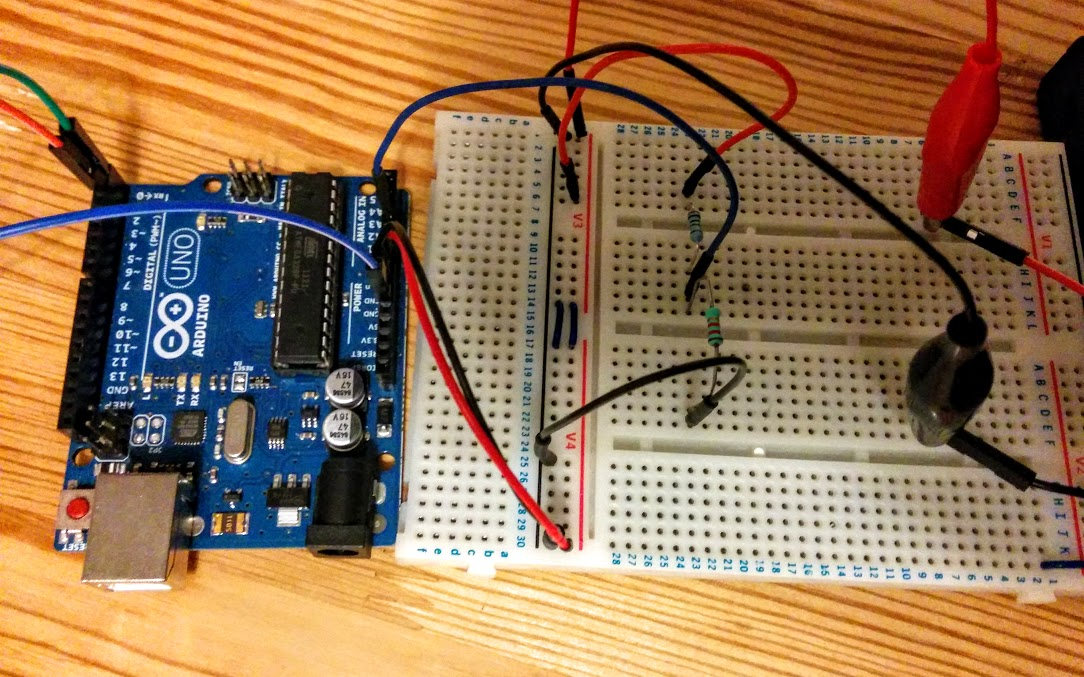# Maker Module 002: Arduino battery charge monitor

Originally posted 2015: information may be out of date, as this tech moves so fast! These 'Maker Modules' were a series of mini 'recipes' for extending components which can in turn be combined into something cool.

This design pattern uses a voltage divider, registering via an analog input pin, in order to enable an Arduino to monitor its own power supply voltage. This is particularly useful if you have a requirement to trigger shutoff when battery charge gets low (e.g. when using a LiPo battery), or else report battery voltage visually on screen in some application.

## Parts

• 2 Resistors (see selection notes, below)
• Arduino
• Battery
• (optional) Something to report the battery voltage in real time, e.g USB/TTL serial convertor [like this] for serial monitoring, or else a display, or series of coloured LEDs, etc.

## Notes

Let’s say you have an Arduino UNO, and wish to use 6 AA batteries to power it. At a nominal 1.25V apiece, this makes a total supply voltage of 9V. The Arduino will happily consume this via its onboard regulator, to create an onboard logic voltage ~5V.

Let’s assume that we’ll use the default (5V) analogue reference voltage on the Arduino. In this case, a voltage of 5V (or higher) on an analog pin will be read using analogRead() as integer value 1024. Equivalently, half this input voltage will report the value 512, and 0V will report the value 0.

In order to get sensible pin readings from our supply voltage, we need to map the input range (in this case 9V to 0V) into that required for logic input (in the case of our chosen reference voltage, 5V to 0V)…

Let’s select a suitable resistor pair to create this ratio. Using a voltage divider calculator, plug in the input (peak supply voltage at 9V), desired output (reference voltage at 5V), and 1Ω for R1. Note the value that the calculator returns for R2. The ratio between R1 and R2 is key to the correct voltage reduction: by inputting 1Ω for R1, R2 is reported (ignoring the unit) as the other half of the key ratio.

In our example case, the perfect ratio for R1:R2 is 1:1.25. Any resistor pair with the same ratio difference as that calculated here, will scale the maximum supply voltage perfectly to the input reference. Resistor values aren’t ranked in a full continuum however, so it can be tricky getting as close to the desired ratio as possible. Armed with a vague sense that ‘low’ resistor values are a source of current wastage, yet high resistances can create ‘noisy’ input, I found a close mapping (9V to 4.9V) where R1:R2 = 1:1.2, by choosing R1=1000Ω and R2=1200Ω.

## Construction

Wire the circuit, including the divider as in the following diagram. The analogue pin on the Arduino takes its voltage reading in between R1 and R2:## Closing remarks

You can use an alternative reference voltage, so long as the resistance ratio maps the supply to an appropriate input voltage. In my smartwatch for example, I’m using 1.1V reference voltage on a 3.3V Arduino Pro Mini, mapping from a LiPo 4.2V peak supply to just a little over the reference voltage (1.125V), using R1=3270Ω and R2=1196Ω. As the input voltage drops below 1.1V, I start to see the ‘real’ battery voltage reported on the tiny screen, and I can make sure that I power down for a recharge as the battery approaches 3.7V.More projects